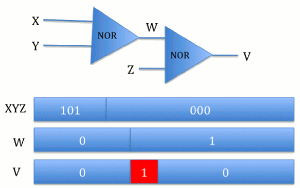# CMOS Power Consumption

## Srikanth Iyer December 1, 2010

### Submitted as coursework for Physics 240, Stanford University, Fall 2010Fig. 1: Two cascaded NOR gates where the change in inputs results in the dynamic hazard shown in red. The power due to thie hazard is wasted.

There are two main components that constitute the power used by a CMOS integrated circuit: static power and dynamic power. Static power essentially consists of the power used when the transistor is not in the process of switching and is essentially determined by the formula

Pstatic = Istatic Vdd

where Vdd is the supply voltage and Istatic is the total current flowing through the device.  Typically, CMOS technology has been praised for its low static power. However, as devices are scaled, gate oxide thicknesses decrease and there is increased probability of tunneling, resulting in larger and larger leakage currents. This subthreshold leakage current is governed by thermodynamics, more specifically the Boltzmann distribution. 

Dynamic power is the sum of transient power consumption (Ptransient) and capacitive load power (Pcap) consumption. Ptransient represents the amount of power consumed when the device changes logic states, i.e. "0" bit to "1" bit or vice versa. Capacitive load power consumption as its name suggests, represents the power used to charge the load capacitance. Together we find that

Pdynamic = Pcap + Ptransient = (CL + C) Vdd2 f N3

where CL is the load capacitance, C is the internal capacitance of the IC, f is the frequency of operation, and N is the number of bits that are switching. What this reveals is that as performance increases, meaning the speed and frequency of the IC increases, the amount of dynamic power also increases. We also see that dynamic power is data dependent and is in fact closely tied to the number of transistors that change states. A more hidden component of dynamic power is loss due to dynamic hazards.

Consider the example in Fig. 1. The red portion of the output represents the dynamic hazard, essentially when the output of the cascaded gates has the "wrong" value during a transition where the output remains the same before and after the inputs change. This power can be calculated using the same formula for static power consumption given in the previous section of this paper. This indicates that glitchy ICs actually consume more power than ICs that do not have such dynamic hazards.

According to Gartner Inc, as of 2008, the number of personal computers installed worldwide had surpassed 1 billion and at a 12 percent annual estimated growth rate, it is expected that the number of PCs will exceed 2 billion in 2014 . According to Intel's official specifications the maximum Thermal Design Power (TDP) for their 2.00 GHz Pentium 4 Processor with 512K Cache is 54.3 W.  By simply multiplying this power value by the number of PCs we can get a rough estimate of the total power CMOS power consumption resulting in a total power of 54.3 GW using the 1 billion worldwide PCs statistic. Note that TDP is not the maximum power consumption of the PC, but rather the maximum power the cooling system is required to dissipate.  This means that the 54.3 GW number is a low estimate for microprocessor power consumption. When we consider that this number only includes personal computers, meaning it does not include the processors in industrial settings, for example Google servers, we see that this estimate is only a fraction of the true world CMOS power consumption.

© Srikanth Iyer. The author grants permission to copy, distribute and display this work in unaltered form, with attribution to the author, for noncommercial purposes only. All other rights, including commercial rights, are reserved to the author.

## References

 C.-T. Sah, Fundamentals of Solid-state Electronics (World Scientific, 1991).

 D. J. Frank, "The Limits of CMOS Scaling from a Power-Constrained Technology Optimization Perspective," Nanohub, 4 Oct 06.

 "Gartner Says More than 1 Billion PCs In Use Worldwide and Headed to 2 Billion Units by 2014," Gartner Inc., 23 Jun 08

 "Intel Pentium 4 Processor 2.00 GHz, 512 Cache, 400 MHz FSB," Intel Corp.

 "Definition of TDP," PC Magazine Encyclopedia.